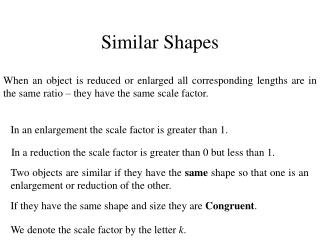Download PresentationSimilar Shapes

# Similar Shapes - PowerPoint PPT PresentationDownload Presentation## Similar Shapes

- - - - - - - - - - - - - - - - - - - - - - - - - - - E N D - - - - - - - - - - - - - - - - - - - - - - - - - - -
##### Presentation Transcript

1. Similar Shapes When an object is reduced or enlarged all corresponding lengths are in the same ratio – they have the same scale factor. In an enlargement the scale factor is greater than 1. In a reduction the scale factor is greater than 0 but less than 1. Two objects are similar if they have the same shape so that one is an enlargement or reduction of the other. If they have the same shape and size they are Congruent. We denote the scale factor by the letter k.

2. a) Find the scale factor in the shapes below. (i) (ii) 3cm 8cm 15cm 12cm Always find the corresponding sides first. (ii) (i)

3. b) In the shape below, determine the scale factor and then the height of the second shape. x cm 1.8cm 2.5cm 10cm

4. Page 16 Exercise 1A Page 17 Exercise 1B

5. Calculations with Similar Shapes In geometry two shapes are similar if one is a scaled version of the other. This means that: (i) Their corresponding angles are equal and (ii) Their corresponding sides are in the same ratio. (same scale factor)

6. a) Are the two shapes below similar? 11.76cm 2.8cm 3.6cm 15.12cm The corresponding sides are: 2.8 and 11.76, 3.6 and 15.12 Since the corresponding sides are in the same ratio and have the same scale factor, the shapes are similar.

7. Page 20 Exercise 3A

8. 60 cm 36cm x cm 80 cm • The two shapes above are similar. Calculate • The scale factor • The width of the smaller object. The 60cm side corresponds with the 36cm side.

9. Page 22 Exercise 3B

10. x y    z b a   c Similar Triangles Triangles are special. If pairs of corresponding angles are equal, then the ratios of lengths of corresponding sides are equal and the triangles are similar. The converse is also true. If the ratios of corresponding sides is equal, then pairs of corresponding angles are equal and the triangles are similar. These triangles are equiangular. So their corresponding sides are in proportion. They have the same scale factor.

11. x  y b a     z c Side a and side x are opposite angle  so they are corresponding sides. This would also be true for sides c and z and sides b and y. Since the scale factor is a constant for those two triangle,

12. 6 4 9 6   8 12  5 3    Triangle are similar if: • they are equiangular • Their corresponding sides have the same ratio. (k) a) Which of the following triangle are similar? (iii) (i) (ii) (v) (iv)

13. 6 4 9 6   8 12  5 3    a) Which of the following triangle are similar? (iii) (i) (ii) (v) (iv) Triangles (ii) and (iv) are equiangular so are similar triangles.

14. Page 25 Exercise 4A Page 26 Exercise 4B

15. 12 15 x 10 Parallel Lines in Triangles A BE is parallel to CD Corresponding angles 12 15 x   E B   D C 10

16. Page 27 Exercise 5

17. T C   7 6   B 8 A  R  9 S Different Positions Similar triangles are not always found in the same position. Calculate the scale factor and the length of the other two sides in triangle RST. RS is opposite angle  AC is opposite angle  Hence AC corresponds to RS

18. 30 V W   (i) Are triangles VWX and XYZ similar? 20 18  X (ii) Find the length of sides s and t.  t s  Z  Y 20 Let us look for equal angles. Vertically Opposite Alternate Angles Hence the triangles are equiangular and are therefore similar.

19. X  X 20 18  s t     W 20 V Z 30 Y Draw the triangles separately to carry out the calculations and to match corresponding sides. The triangle with the unknown sides is usually drawn second

20. Worksheet 1

21. 6 3 3 6 Similar Areas Here we have two similar shapes. It is obvious that the scale factor is 2 This type of scale factor is known as the linear scale factor. Area shape 1 = Area shape 2 = Area Scale Factor: Area scale factor =

22. To find the area scale factor: • find the linear scale factor k. • Square the linear scale factor k2 a) Determine the area of the second shape if both shapes are similar. 8cm2 x cm2 6cm 8cm Area Scale factor: Linear scale factor: Area of second shape

23. If a shape is enlarged or reduced by a scale factor k, the area is enlarged or reduced by a scale factor equal to k2 Worksheet 2

24. Similar Volumes When a figure is enlarged or reduced by a scale factor k, the volume of the figure is enlarged or reduced by a scale factor k3. Scale factor: 1.5 ltrs 40cm ? ltrs 20cm Volume in larger shape Volume scale factor

25. Worksheet 3